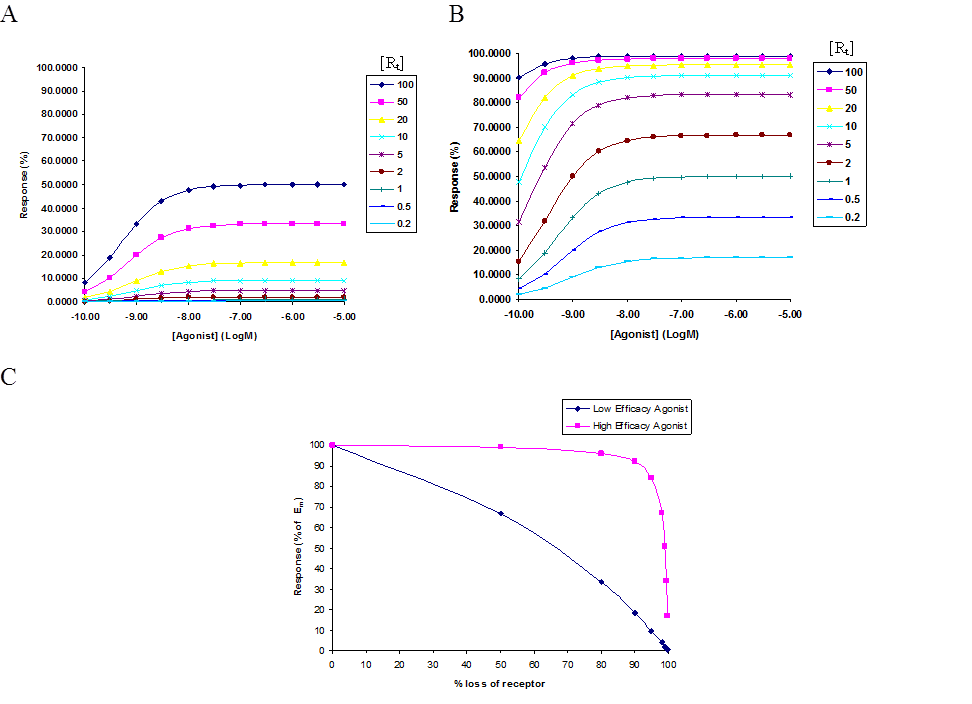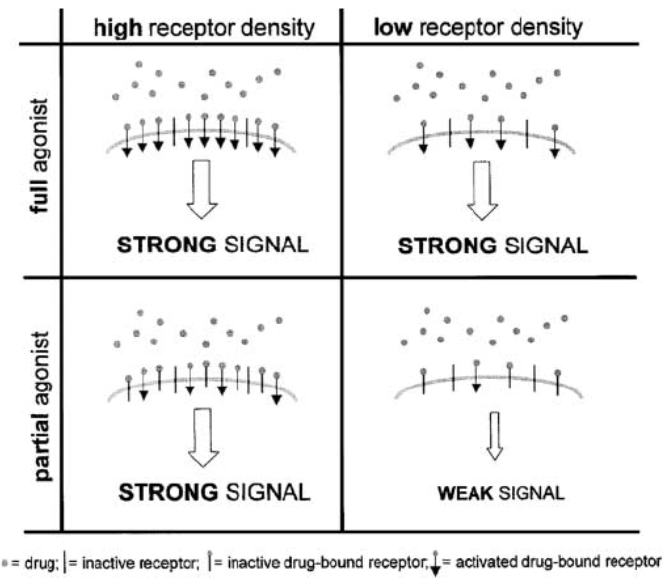# Black and Leff Operational model of Efficacy

This modified definition of receptor occupancy defined by the del Castillo-Katz model now allows us to re-define R. P. Stephenson’s original postulate in Eq. (1.0) as follows:$Stimulus (SA) = e^*_A \delta_{occupied(A)} = S = e^*_A \frac {[A]}{K_{effective} + [A]}$                                     (1.29)

Here,$e^*$ is defined as the function that provides a link between the occupancy of the receptor (AR), and the observed effect, S. In 1983, Black and Leff (Black and Leff 1983) proposed a rectangular hyperbolic relationship between this effect$e^*$  as a function of receptor occupancy AR, represented as follows:$\frac{E}{E_m} = \frac{[AR]}{K_E + [AR]}$                                                       (1.30)

Where$E_m$, is the maximal effect of an agonist in a system and$K_E$ is the value of [AR] that elicits half the maximal effect.

(Note: This particular treatment of E differs in meaning from that used in the del Castillo-Katz Model)

Since [AR] is defined, by the Hill-Langmuir hyperbolic isotherm as:$[AR] = \frac {[R_t][A]}{K_A + [A]}$

Where$[R_t]$ is the total receptor concentration and$K_A$ is the association constant for the agonist, we can combine this into equation 1.30 to give the following relationship:$\frac {E}{E_m} = \frac {[R_t][A]}{K_A K_E + ([R_t] + [K_E])[A]}$                                           (1.31)

And unlike the del Castillo-Katz derivation, which allows us to quantitatively measure the intrinsic efficacy of an agonist defined by the$\frac {E}{1+E}$ parameter; the Black and Leff model of Operational Efficacy allows us to accurately quantify the effect of receptor reserve in a given system. Equation 1.31 can be further simplified by dividing the numerator and the denominator by$K_E$ to obtain the following:$\frac {E}{E_m} = \frac{\frac{[R_t][A]}{K_E}}{\frac{K_A K_E}{K_E} + \frac {[R_t][A]}{K_E} + \frac {K_E [A]}{K_E}}$ which gives$\frac {E}{E_m} = \frac{\frac {[R_t][A]}{K_E}}{K_A + \frac{[R_t][A]}{K_E} + [A]}$

Or,$E = \frac {E_m \tau [A]}{K_A + [A] + \tau[A]}$                                           (1.32)

Where$\tau = \frac{[R_t]}{K_E}$                                                                      (1.33)

This$(\tau)$ ratio, allows us to experimentally quantify the relationship between the potency of an agonist, with regards to eliciting a desired functional response, as determined by the affinity value ($K_E$), to the efficiency of the receptor to translate a given level of receptor occupancy to response.Figure 1. Concentration response theoretical curves generated based on the Black and Leff model of Operational Efficacy. The response is shown as a function of receptor reserve of a system where the affinity of the agonist,$K_A$, was fixed at 1µM and$[R_t]$ was titrated from 100 to 0.1. (A) Simulates the response with a low efficacy agonist, with$K_E$ = 100. (B) Simulates response with a high efficacy agonist, with$K_E$ = 1. (C) Simulates the effect on maximal response, by a low and high efficacy agonist, as a result of receptor loss. (Also, represented in Charlton 2009)

A closer look at the isomerization constant E, in the del Castillo-Katz scheme shows us that E is, in fact, a reciprocal of the$K_E$ value defined by Black and Leff where they are both effectively measuring the ability of the agonist to stabilize the active conformation of the receptor [AR*]. Therefore, substituting$E = \frac {1}{K_E}$ into equation 1.26, we can get a similar quantitative measurement of receptor occupancy and response from the del Castillo-Katz derivation as follows:$\delta_{occupied} = (\frac {[A]}{\frac {K_A}{1 + E} + [A]})$, where$\delta_{occupied} = \delta_{AR} + \delta_{AR^*}$

Written, with the substitution for E,$\delta_{occupied} = (\frac {[A]}{\frac{K_A}{1 + \frac {1}{K_E}} + [A]}) = \frac {[A]}{K_A K_E + [A] K_E + [A]}$

It follows that,$AR + AR^* = \frac {[A][R_t]}{K_A K_E + [A] K_E + [A]}$

Further dividing the numerator and denominator by$K_E$ would yield:$AR + AR^* = \frac{\frac{[A][R_t]}{K_E}}{\frac{K_A K_E + [A] K_E + [A]}{K_E}}$ or$\frac{[A]\tau}{K_A + [A] + \frac{[A]}{K_E}}$

Rewritten as,$AR + AR^* = \frac{\tau[A]}{K_A + [A] + \frac{\tau [A]}{[R_t]}}$                                                       (1.34)

Both equations 1.32 and 1.34, point to a more complex relationship between receptor occupancy and response. It stands to reason, looking at this relationship, that a partial agonist, with full receptor occupancy, would still elicit a sub maximal response given its inherently low intrinsic efficacy for the receptor and further, that this could be corrected, by increasing the number of total receptors in the system so that a larger fraction of receptor population is activated thus compensating for the low intrinsic activity of the partial agonist (Figure 3) (Charlton 2009). In fact, in systems where a desired response is required to be both rapid in its onset, as well as, rapid in termination, this can be very easily achieved by using low affinity (i.e. low KA) agonists at very low concentrations by improving their sensitivity to response through an increased concentration of receptors within the system. This has been very clearly demonstrated by the use of heterologous expression systems where the efficacy of a a2A-adrenoceptor partial agonist was improved by a left-shifted EC50 and a significant potentiation of the maximal response for agonist elicitied MAP kinase activation in systems differentially expressing recombinant a2A-adrenoceptor (Tan et al. 2002).

This relationship between a drug’s intrinsic efficacy, receptor reserve and the degree of stimulus can be summed up by the schematic below:(Figure taken from Hanania, N. A., et. al., 2002)

Here the effect of functional efficacy is shown to be clearly dependent on the size of the spare receptor population in the system. In conditions where [A] >>$[R_t]$, the degree of receptor occupancy is very high and roughly equivalent between a full and a partial agonist, the left column shows that the differences between a partial agonist and a full agonist are hardly discernable in a system with an excess of spare receptors and the response is less dependent on the inherent ‘intrinsic efficacy’ of the agonist itself. This is in contrast to conditions on the right column where the receptor density is limiting. Here, given full receptor occupancy, a full agonist would manage to elicit the maximal response$(E_m)$; however, a partial agonist would elicit its response which is a function of its intrinsic efficacy as defined by$\frac{E}{1+E}$ parameter, or in other words, the response is determined by the operational parameter tau$(\tau)$ which would yield a weaker signal, in comparison to the full agonist. Thus, it could be inferred that the degree of stimulus can reflect the differential levels of receptor reserve in different tissues.